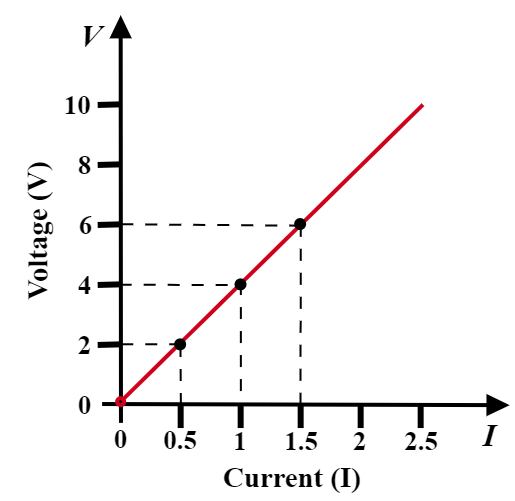# (a) State the relation correlating the electric current flowing in a conductor and the voltage applied across it. Also, draw a graph to show this relationship.(b) Find the resistance of a conductor if the electric current flowing through it is 0.35 A when the potential difference across it is 1.4 V.

(a) If the physical conditions in a conductor remain unchanged (such as temperature), then the electric current flowing through the conductor is directly proportional to the applied potential difference across the two ends of it.

The graph given below depicts the relation between potential difference and current.(b) Given:

Current, $I=0.35A$

Potential difference, $V=1.4V$

To find: Resistance of conductor, $R$.

Solution:

From Ohm's Law, we know that-

$V=I\times R$

For resistance, $R$ it can be rearranged as-

$R=\frac {V}{I}$

Substituting the given values, we get-

$R=\frac {1.4}{0.35}$

$R=\frac {14\times 100}{35\times10}$

$R=\frac {140}{35}$

$R=4\Omega$

Thus, the resistance of conductor, $R$ is 4 Ohm.

Updated on: 10-Oct-2022

229 Views